### Example 1

In the following example, there are two classification variables, A and B , with two and three values, respectively. Regressors for a one-way model (the default model order) are generated using the the default dummy method. The five regressors generated are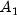,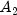,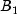,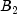and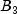.
```
import com.imsl.stat.*;
import com.imsl.math.PrintMatrix;

public class RegressorsForGLMEx1 {

public static void main(String args[]) {
double x[][] = {
{10.0, 5.0},
{20.0, 15.0},
{20.0, 10.0},
{10.0, 10.0},
{10.0, 15.0},
{20.0, 5.0}
};

RegressorsForGLM r = new RegressorsForGLM(x, 2);
double regressors[][] = r.getRegressors();
int n = r.getNumberOfRegressors();
System.out.println("Number of regressors = " + n);
new PrintMatrix("Regressors").print(regressors);
}
}
```

#### Output

```Number of regressors = 5
Regressors
0  1  2  3  4
0  1  0  1  0  0
1  0  1  0  0  1
2  0  1  0  1  0
3  1  0  0  1  0
4  1  0  0  0  1
5  0  1  1  0  0

```